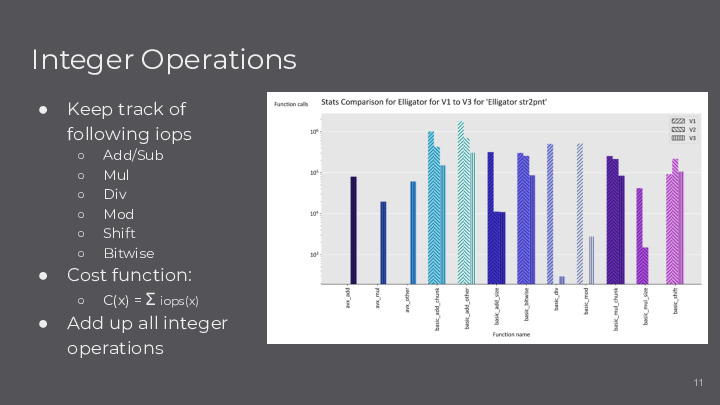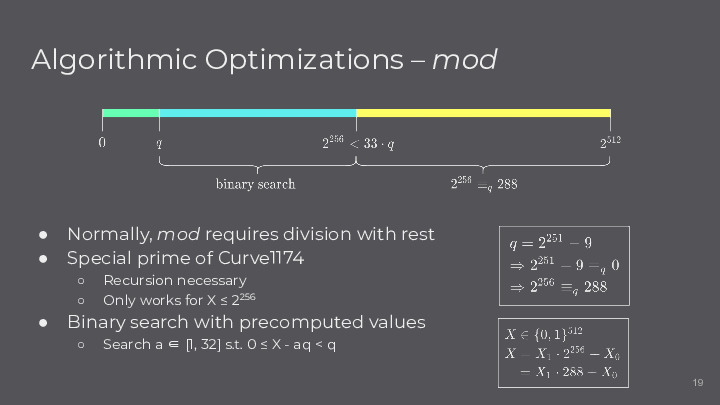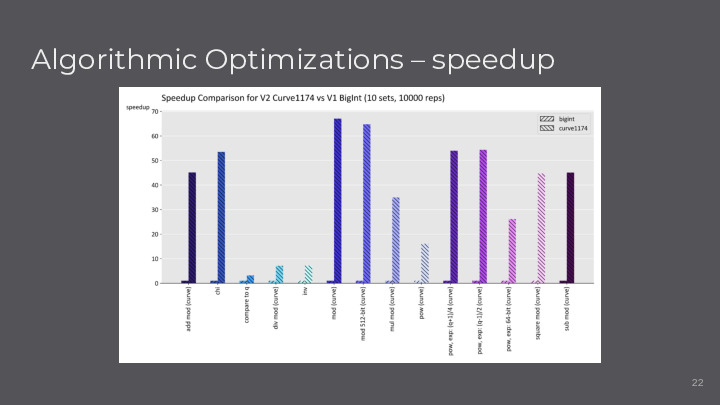# Optimizing Elligator 1 on Curve1174

Date:

At the end of the Advanced Systems Lab, we presented a brief overview on our optimizations for Elligator 1, described in this blog post

## HighlightsWe tracked single integer operations in our implementation by tagging them in the source code. The bar plot on the right shows that we were able to reduce some operations, such as divisions, significantly during the optimization steps from V1 (naive implementation) to V2 (standard optimizations) and V3 (vector optimizations).This slide explains one of our major optimizations. Since the modulus q of Curve1174 is close to 2^256, we can replace 2^256 by 288 modulo q. Therefore, we can split blocks that are larger than 256 bits (say two chunks X1 and X0 that are both 256 bits, such that X1 * 2^256 + X0 is a 512-bit integer) into the sum of two 256-bit integers and a multiplication with the constant 288. Moreover, for numbers q < X <= 2^256, we notice that there are only 32 intervals of length q in that area, so we precompute all boundary values and perform binary search among them. More details are described in the paper.This figure gives an overview on the speedups that we achieved with standard optimizations (V2) compared to the naive implementation (V1). Please look at the paper for the various techniques that we applied.

## Full Presentation

The presentation slides are available here.